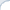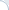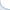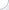About one year ago, a Quanta magazine published an article on the universal method to sort complex information. It is concerned with a theoretical work on solving a nearest-neighbor search problem for various metric distances. Even more precisely, this work attempts to answer a question about what kind of a distance metric permits an efficient nearest neighbor search. Though this is surely an important and solid theoretical work, the Quanta magazine completely ignores the fact that from the practical perspective this problem has satisfactory solutions. Not only existing methods work for metric distances, good results can often be obtained for weird non-metric dissimilarities (or distances as we like to call them). Sometimes, they work when these distances are even non-symmetric!

Are these methods universal? They certainly are not, but nothing is universal in nearest-neighbor search. There are challenging data sets, which cannot be searched efficiently even with the Euclidean distance! This issue as well as the history of non-metric k-NN search is briefly surveyed in my thesis. However, in some cases we can do really well by using a tree-based or a neighborhood-graph based approaches. In my thesis, I carried out a series of evaluations to verify this. I am pleased that all of the main results are now formally published, in particular, including two recent SISAP papers:

1. Boytsov, L., Nyberg. E., 2019. Accurate and Fast Retrieval for Complex Non-metric Data via Neighborhood Graphs.

2. Boytsov, L., Nyberg. E., 2019. Pruning Algorithms for Low-Dimensional Non-metric k-NN Search: A Case Study.

I think these papers are concerned with important research questions and I am going to briefly highlight results.

Neighborhood-graphs is a class of new-old methods, which delivers state of the art results on many data sets. However, little is known how they behave on non-symmetric distances. We were probably the first to test them on non-symmetric distances such as KL-divergence [1, 2]. Turns out, however, these tests relied on data sets that were only mildly non-symmetric. In the follow-up work, we have really stress tested them and discovered the following:

1. It is never a good idea to deal with non-symmetric distances by symmetrizing the distance first and using the symmetrized distance as a part of a filter-and-refine pipeline.

2. However, it is not even necessary. In many cases, indeed, neighborhood-graphs deliver state-of-the-art performance out of the box.

3. Importantly, one has to be consistent in the order of distance function arguments (although there are exceptions as I describe below). If the indexing procedure relies on a different order (e.g., by mistake), the results could be disastrous (I have made this mistake and it cost me a lot of time).

4. That said, using a different distance function at index time can produce sometimes better results. Again this is not a universal property. One somewhat obvious choice of possibly better index-time distance function is a symmetrized variant of the original distance. Quite surprisingly, the argument-reversed distance can deliver good results too, but, as I explain above, the results can be disastrous for some other datasets and distances. I think this discovery begs a research question: what is the optimal distance-time function?

Although graph-based retrieval is state-of-the-art for high-dimensional data it can be an overkill for low-dimensional data, where tree-based approaches can work really well. In particular, we compare two approaches to adapt standard metric tree methods to non-metric similarities. One is the effective piecewise-linear modification of the pruning rule, which we published at NIPS in 2013. In fact, for the Euclidean distance, it is as efficient as the classic projection-based LSH. However, due to the linear nature of the approximation, it is sometimes not a good fit for non-metric dissimilarities. In contrast, Tomas Skopal TriGen algorithm can be better in this case.

TriGen is an ingenious algorithm that finds a monotonic distance transformation that makes a dissimilarity look more like metric. However, TriGen has two drawbacks: it does not work out of the box with non-symmetric distances and its implementation of the distance-modifying transformation can be a bit expensive. What we show is that, perhaps, the best solution is a hybrid: First, we can apply a cheap concave (or near concave) distance transformation such as the square root. Second, we can fit a piecewise-linear decision function for this transformed distance.

In conclusion, I want to emphasize that, although nearest-neighbor search has no universal solution, there are a number of working general-distance approaches. Some good solutions are implemented in NMSLIB, which is the first generic library for metric and non-metric k-NN search.